# RD Sharma solutions for Class 10 Maths chapter 7 - Triangles [Latest edition]

#### Chapters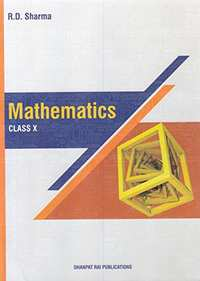## Chapter 7: Triangles

Exercise 7.1Exercise 7.2Exercise 7.3Exercise 7.4Exercise 7.5Exercise 7.6Exercise 7.7Exercise 7.8Exercise 7.9Exercise 7.10
Exercise 7.1 [Pages 2 - 3]

### RD Sharma solutions for Class 10 Maths Chapter 7 Triangles Exercise 7.1 [Pages 2 - 3]

Exercise 7.1 | Q 1.1 | Page 2

Fill in the blanks using correct word given

All circles are __________.

• congruent

• similar

Exercise 7.1 | Q 1.2 | Page 2

Fill in the blanks using correct word given

All squares are __________.

• similar

• congruent

Exercise 7.1 | Q 1.3 | Page 2

Fill in the blanks using correct word given

All __________ triangles are similar.

• isosceles

• equilateral

Exercise 7.1 | Q 1.4 | Page 2

Two triangles are similar, if their corresponding angles are .......... (proportional, equal)

Exercise 7.1 | Q 1.5 | Page 2

Two triangles are similar, if their corresponding sides are .......... (proportional, equal)

Exercise 7.1 | Q 1.6 | Page 2

Fill in the blanks using correct word given in the brackets:−

Two polygons of the same number of sides are similar, if (a) their corresponding angles are __________ and (b) their corresponding sides are __________. (equal, proportional)

Exercise 7.1 | Q 2.1 | Page 3

Write the truth value (T/F) of each of the following statement

Any two similar figures are congruent.

Exercise 7.1 | Q 2.2 | Page 3

Write the truth value (T/F) of each of the following statement

Any two congruent figures are similar.

Exercise 7.1 | Q 2.3 | Page 3

Write the truth value (T/F) of each of the following statement

Two polygons are similar, if their corresponding sides are proportional.

Exercise 7.1 | Q 2.4 | Page 3

Write the truth value (T/F) of each of the following statement

Two polygons are similar if their corresponding angles are proportional.

Exercise 7.1 | Q 2.5 | Page 3

Write the truth value (T/F) of each of the following statement

Two triangles are similar if their corresponding sides are proportional.

Exercise 7.1 | Q 2.6 | Page 3

Write the truth value (T/F) of each of the following statement

Two triangles are similar if their corresponding angles are proportional.

Exercise 7.2 [Pages 19 - 31]

### RD Sharma solutions for Class 10 Maths Chapter 7 Triangles Exercise 7.2 [Pages 19 - 31]

Exercise 7.2 | Q 1.01 | Page 19

In ΔABC, D and E are points on the sides AB and AC respectively such that DE || BC

If AD = 6 cm, DB = 9 cm and AE = 8 cm, find AC.

Exercise 7.2 | Q 1.02 | Page 19

In ΔABC, D and E are points on the sides AB and AC respectively such that DE || BC

If "AD"/"DB"=3/4 and AC = 15 cm, find AE

Exercise 7.2 | Q 1.03 | Page 19

In ΔABC, D and E are points on the sides AB and AC respectively such that DE || BC

If "AD"/"DB"=2/3 and AC = 18 cm, find AE

Exercise 7.2 | Q 1.04 | Page 19

In ΔABC, D and E are points on the sides AB and AC respectively such that DE || BC

If AD = 4, AE = 8, DB = x – 4, and EC = 3x – 19, find x.

Exercise 7.2 | Q 1.05 | Page 19

In ΔABC, D and E are points on the sides AB and AC respectively such that DE || BC

If AD = 8cm, AB = 12 cm and AE = 12 cm, find CE.

Exercise 7.2 | Q 1.06 | Page 19

In ΔABC, D and E are points on the sides AB and AC respectively such that DE || BC

If AD = 4 cm, DB = 4.5 cm and AE = 8 cm, find AC.

Exercise 7.2 | Q 1.07 | Page 19

In ΔABC, D and E are points on the sides AB and AC respectively such that DE || BC

If AD = 2 cm, AB = 6 cm and AC = 9 cm, find AE.

Exercise 7.2 | Q 1.08 | Page 19

In ΔABC, D and E are points on the sides AB and AC respectively such that DE || BC

If "AD"/"BD"=4/5 and EC = 2.5 cm, find AE

Exercise 7.2 | Q 1.09 | Page 19

In ΔABC, D and E are points on the sides AB and AC respectively such that DE || BC

If AD = x, DB = x − 2, AE = x + 2 and EC = x − 1, find the value of x.

Exercise 7.2 | Q 1.1 | Page 19

In ΔABC, D and E are points on the sides AB and AC respectively such that DE || BC

If AD = 8x − 7, DB = 5x − 3, AE = 4x − 3 and EC = (3x − 1), find the value of x.

Exercise 7.2 | Q 1.11 | Page 19

In ΔABC, D and E are points on the sides AB and AC respectively such that DE || BC

If AD = 4x − 3, AE = 8x – 7, BD = 3x – 1 and CE = 5x − 3, find the volume of x.

Exercise 7.2 | Q 1.12 | Page 19

In ΔABC, D and E are points on the sides AB and AC respectively such that DE || BC

If AD = 2.5 cm, BD = 3.0 cm and AE = 3.75 cm, find the length of AC.

Exercise 7.2 | Q 2.1 | Page 19

In a ΔABC, D and E are points on the sides AB and AC respectively. For  the following case show that DE || BC

AB = 2cm, AD = 8cm, AE = 12 cm and AC = l8cm.

Exercise 7.2 | Q 2.2 | Page 19

In a ΔABC, D and E are points on the sides AB and AC respectively. For  the following case show that DE || BC

AB = 5.6cm, AD = 1.4cm, AC= 7.2 cm and AE = 1.8 cm.

Exercise 7.2 | Q 2.3 | Page 19

In a ΔABC, D and E are points on the sides AB and AC respectively. For  the following case show that DE || BC

AB = 10.8 cm, BD = 4.5 cm, AC = 4.8 cm and AE = 2.8 cm.

Exercise 7.2 | Q 2.4 | Page 19

In a ΔABC, D and E are points on the sides AB and AC respectively. For  the following case show that DE || BC

AD = 5.7 cm, BD = 9.5 cm, AE = 3.3 cm and EC = 5.5 cm.

Exercise 7.2 | Q 3 | Page 19

In a ΔABC, P and Q are points on sides AB and AC respectively, such that PQ || BC. If AP = 2.4 cm, AQ = 2 cm, QC = 3 cm and BC = 6 cm, find AB and PQ.

Exercise 7.2 | Q 4 | Page 19

In a ΔABC, D and E are points on AB and AC respectively such that DE || BC. If AD = 2.4cm, AE = 3.2 cm, DE = 2cm and BC = 5 cm, find BD and CE.

Exercise 7.2 | Q 5 | Page 19

In below Fig., state if PQ || EF.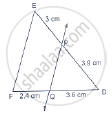Exercise 7.2 | Q 6

M and N are points on the sides PQ and PR respectively of a ΔPQR. For the following case, state whether MN || QR

PM = 4cm, QM = 4.5 cm, PN = 4 cm and NR = 4.5 cm

Exercise 7.2 | Q 7

In three line segments OA, OB, and OC, points L, M, N respectively are so chosen that LM || AB and MN || BC but neither of L, M, N nor of A, B, C are collinear. Show that LN ||AC.

Exercise 7.2 | Q 8 | Page 31

If D and E are points on sides AB and AC respectively of a ΔABC such that DE || BC and BD = CE. Prove that ΔABC is isosceles.

Exercise 7.3 [Pages 31 - 32]

### RD Sharma solutions for Class 10 Maths Chapter 7 Triangles Exercise 7.3 [Pages 31 - 32]

Exercise 7.3 | Q 1.1 | Page 31

In a ΔABC, AD is the bisector of ∠A, meeting side BC at D.

If BD = 2.5cm, AB = 5cm and AC = 4.2cm, find DC.

Exercise 7.3 | Q 1.2 | Page 31

In a ΔABC, AD is the bisector of ∠A, meeting side BC at D.

If BD = 2cm, AB = 5cm and DC = 3cm, find AC.

Exercise 7.3 | Q 1.3 | Page 31

In a ΔABC, AD is the bisector of ∠A, meeting side BC at D.

If AB = 3.5 cm, AC = 4.2 cm and DC = 2.8 cm, find BD.

Exercise 7.3 | Q 1.4 | Page 31

In a ΔABC, AD is the bisector of ∠A, meeting side BC at D.

If AB = 10 cm, AC =14 cm and BC =6 cm, find BD and DC.

Exercise 7.3 | Q 1.5 | Page 31

In a ΔABC, AD is the bisector of ∠A, meeting side BC at D.

If AC = 4.2 cm, DC = 6 cm and 10 cm, find AB

Exercise 7.3 | Q 1.6 | Page 31

In a ΔABC, AD is the bisector of ∠A, meeting side BC at D.

If AB = 5.6 cm, AC = 6cm and DC = 3cm, find BC.

Exercise 7.3 | Q 1.7 | Page 31

In a ΔABC, AD is the bisector of ∠A, meeting side BC at D.

If AD = 5.6 cm, BC = 6cm and BD = 3.2 cm, find AC.

Exercise 7.3 | Q 1.8 | Page 31

In a ΔABC, AD is the bisector of ∠A, meeting side BC at D.

If AB = 10cm, AC = 6 cm and BC = 12 cm, find BD and DC.

Exercise 7.3 | Q 2 | Page 31

In the following Figure, AE is the bisector of the exterior ∠CAD meeting BC produced in E. If AB = 10cm, AC = 6cm and BC = 12 cm, find CE.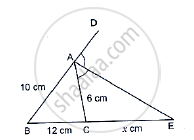Exercise 7.3 | Q 3 | Page 31

In the following Figure, ΔABC is a triangle such that "AB"/"AC"="BD"/"DC", ∠B = 70°, ∠C = 50°. Find ∠BAD.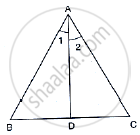Exercise 7.3 | Q 4.1 | Page 31

In the following figure, check whether AD is the bisector of ∠A of ∆ABC in the following case:

AB = 5 cm, AC = 10 cm, BD = 1.5 cm and CD = 3.5 cm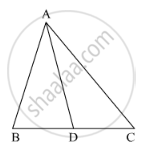Exercise 7.3 | Q 4.2 | Page 31

In the following figure, check whether AD is the bisector of ∠A of ∆ABC in the following case:

AB = 4 cm, AC = 6 cm, BD = 1.6 cm and CD = 2.4 cmExercise 7.3 | Q 4.3 | Page 31

In the following figure, check whether AD is the bisector of ∠A of ∆ABC in the following case:

AB = 8 cm, AC = 24 cm, BD = 6 cm and BC = 24 cmExercise 7.3 | Q 4.4 | Page 31

In the following figure, check whether AD is the bisector of ∠A of ∆ABC in the following case:

AB = 6 cm, AC = 8 cm, BD = 1.5 cm and CD = 2 cmExercise 7.3 | Q 4.5 | Page 31

In the following figure, check whether AD is the bisector of ∠A of ∆ABC in the following case:

AB =  5 cm, AC = 12 cm, BD = 2.5 cm and BC = 9 cmExercise 7.3 | Q 6 | Page 32

In ΔABC , if ∠1 = ∠2, prove that "AB"/"AC"="BD"/"DC"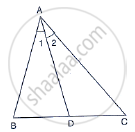Exercise 7.3 | Q 7 | Page 32

D, E and F are the points on sides BC, CA and AB respectively of ΔABC such that AD bisects ∠A, BE bisects ∠B and CF bisects ∠C. If AB = 5 cm, BC = 8 cm and CA = 4 cm, determine AP, CE and BD.

Exercise 7.4 [Page 37]

### RD Sharma solutions for Class 10 Maths Chapter 7 Triangles Exercise 7.4 [Page 37]

Exercise 7.4 | Q 1.1 | Page 37

In below figure, If AB || CD, find the value of x.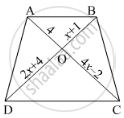Exercise 7.4 | Q 1.2 | Page 37

In the below figure, If AB || CD, find the value of x.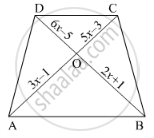Exercise 7.4 | Q 1.3 | Page 37

In below figure, AB || CD. If OA = 3x – 19, OB = x – 4, OC = x – 3 and OD = 4, find x.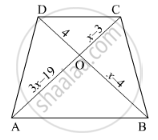Exercise 7.5 [Pages 73 - 76]

### RD Sharma solutions for Class 10 Maths Chapter 7 Triangles Exercise 7.5 [Pages 73 - 76]

Exercise 7.5 | Q 1 | Page 73

In figure, ∆ACB ~ ∆APQ. If BC = 8 cm, PQ = 4 cm, BA = 6.5 cm, AP = 2.8 cm, find CA and AQ.

Exercise 7.5 | Q 2 | Page 73

In the following figure, AB || QR. Find the length of PB.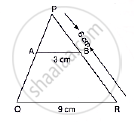Exercise 7.5 | Q 3 | Page 73

In the following figure, XY || BC. Find the length of XY.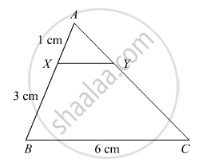Exercise 7.5 | Q 4 | Page 73

In a right angled triangle with sides a and b and hypotenuse c, the altitude drawn on the hypotenuse is x. Prove that ab = cx.

Exercise 7.5 | Q 5 | Page 74

In the following Figure, ∠ABC = 90° and BD ⊥ AC. If BD = 8 cm and AD = 4 cm, find CD.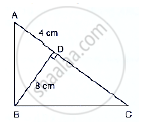Exercise 7.5 | Q 6 | Page 74

In the following Figure, ∠ABC = 90° and BD ⊥ AC. If AB = 5.7 cm, BD = 3.8 cm and CD = 5.4 cm, find BC.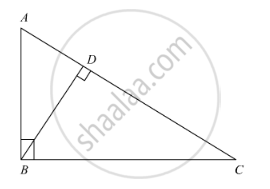Exercise 7.5 | Q 7 | Page 74

In the following Figure, DE || BC such that AE = (1/4) AC. If AB = 6 cm, find AD.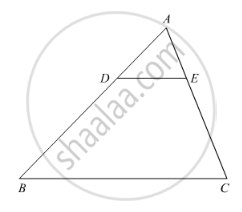Exercise 7.5 | Q 9 | Page 76

In the following figure, PA, QB and RC are each perpendicular to AC. Prove that 1/x+1/z=1/y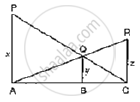Exercise 7.5 | Q 9 | Page 74

Diagonals AC and BD of a trapezium ABCD with AB || DC intersect each other at the point O. Using similarity criterion for two triangles, show that "OA"/"OC"="OB"/"OD"

Exercise 7.5 | Q 10 | Page 74

In the following figure, ABC and AMP are two right triangles, right-angled at B and M respectively, prove that: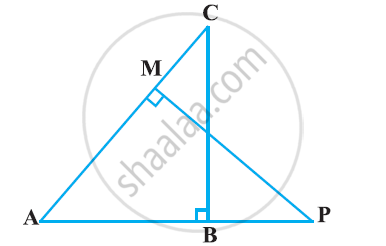1. ΔABC ~ ΔAMP
2. ("CA")/("PA") = ("BC")/("MP")
Exercise 7.5 | Q 10 | Page 74

In the following figure, ABC and AMP are two right triangles, right-angled at B and M respectively, prove that:("CA")/("PA") = ("BC")/("MP")

Exercise 7.5 | Q 12 | Page 75

In below figure, ∠A = ∠CED, Prove that ΔCAB ~ ΔCED. Also, find the value of x.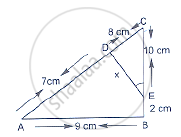Exercise 7.5 | Q 13 | Page 75

The perimeters of two similar triangles are 25 cm and 15 cm respectively. If one side of first triangle is 9 cm, what is the corresponding side of the other triangle?

Exercise 7.5 | Q 14 | Page 75

In ΔABC and ΔDEF, it is being given that: AB = 5 cm, BC = 4 cm and CA = 4.2 cm; DE=10cm, EF = 8 cm and FD = 8.4 cm. If AL ⊥ BC and DM ⊥ EF, find AL: DM.

Exercise 7.5 | Q 15 | Page 75

D and E are the points on the sides AB and AC respectively of a ΔABC such that: AD = 8 cm, DB = 12 cm, AE = 6 cm and CE = 9 cm. Prove that BC = 5/2 DE.

Exercise 7.5 | Q 16 | Page 75

D is the mid-point of side BC of a ΔABC. AD is bisected at the point E and BE produced cuts AC at the point X. Prove that BE : EX = 3 : 1

Exercise 7.5 | Q 17 | Page 75

ABCD is a parallelogram and APQ is a straight line meeting BC at P and DC produced at Q. Prove that the rectangle obtained by BP and DQ is equal to the AB and BC.

Exercise 7.5 | Q 18

ABCD is a quadrilateral in which AD = BC. If P, Q, R, S be the mid-points of AB, AC, CD and BD respectively, show that PQRS is a rhombus.

Exercise 7.5 | Q 18 | Page 75

In ΔABC, AL and CM are the perpendiculars from the vertices A and C to BC and AB respectively. If AL and CM intersect at O, prove that:

(i) ΔOMA and ΔOLC

(ii) "OA"/"OC"="OM"/"OL"

Exercise 7.5 | Q 19

In Figure below, if AB ⊥ BC, DC ⊥ BC and DE ⊥ AC, Prove that Δ CED ~ ABC.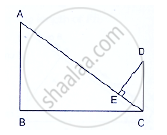Exercise 7.5 | Q 20 | Page 75

In an isosceles ΔABC, the base AB is produced both the ways to P and Q such that AP × BQ = AC2. Prove that ΔAPC ~ ΔBCQ.

Exercise 7.5 | Q 21 | Page 75

A girl of height 90 cm is walking away from the base of a lamp-post at a speed of 1.2m/sec. If the lamp is 3.6 m above the ground, find the length of her shadow after 4 seconds.

Exercise 7.5 | Q 22 | Page 75

A vertical stick of length 6 m casts a shadow 4 m long on the ground and at the same time a tower casts a shadow 28 m long. Find the height of the tower.

Exercise 7.5 | Q 23 | Page 75

In below Figure, ΔABC is right angled at C and DE ⊥ AB. Prove that ΔABC ~ ΔADE and Hence find the lengths of AE and DE.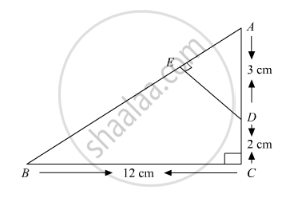Exercise 7.5 | Q 25 | Page 76

In Fig below we have AB || CD || EF. If AB = 6 cm, CD = x cm, EF = 10 cm, BD = 4 cm and DE = y cm, calculate the values of x and y.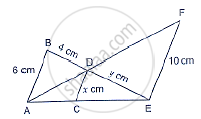Exercise 7.6 [Pages 94 - 96]

### RD Sharma solutions for Class 10 Maths Chapter 7 Triangles Exercise 7.6 [Pages 94 - 96]

Exercise 7.6 | Q 1.1 | Page 94

If ∆ABC ~ ∆DEF such that area of ∆ABC is 16cm2 and the area of ∆DEF is 25cm2 and BC = 2.3 cm. Find the length of EF.

Exercise 7.6 | Q 1.2 | Page 94

If ∆ABC is similar to ∆DEF such that ∆DEF = 64 cm2 , DE = 5.1 cm and area of ∆ABC = 9 cm2 . Determine the area of AB

Exercise 7.6 | Q 1.3 | Page 94

Triangles ABC and DEF are similar If AC = 19cm and DF = 8 cm, find the ratio of the area of two triangles.

Exercise 7.6 | Q 1.4 | Page 94

Triangles ABC and DEF are similar If area (ΔABC) = 36 cm2, area (ΔDEF) = 64 cm2 and DE = 6.2 cm, find AB.

Exercise 7.6 | Q 1.5 | Page 94

Triangles ABC and DEF are similar If AB = 1.2 cm and DE = 1.4 cm, find the ratio of the areas of ΔABC and ΔDEF.

Exercise 7.6 | Q 2 | Page 95

In figure below ΔACB ~ ΔAPQ. If BC = 10 cm, PQ = 5 cm, BA = 6.5 cm and AP = 2.8 cm,
find CA and AQ. Also, find the area (ΔACB): area (ΔAPQ)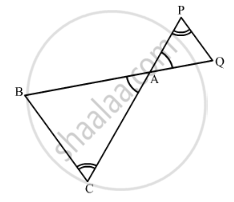Exercise 7.6 | Q 3 | Page 95

The areas of two similar triangles are 81 cm2 and 49 cm2 respectively. Find the ratio of their corresponding heights. What is the ratio of their corresponding medians?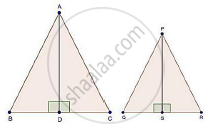Exercise 7.6 | Q 4 | Page 95

The areas of two similar triangles are 169 cm2 and 121 cm2 respectively. If the longest side of the larger triangle is 26 cm, find the longest side of the smaller triangle.

Exercise 7.6 | Q 5 | Page 95

The areas of two similar triangles are 25 cm2 and 36 cm2 respectively. If the altitude of the first triangle is 2.4 cm, find the corresponding altitude of the other.

Exercise 7.6 | Q 6 | Page 95

The corresponding altitudes of two similar triangles are 6 cm and 9 cm respectively. Find the ratio of their areas.

Exercise 7.6 | Q 7 | Page 95

ABC is a triangle in which ∠A =90°, AN⊥ BC, BC = 12 cm and AC = 5cm. Find the ratio of the areas of ΔANC and ΔABC.

Exercise 7.6 | Q 8.1 | Page 95

In Figure, DE || BC If DE = 4 cm, BC = 6 cm and Area (ΔADE) = 16 cm2, find the area of ΔABC.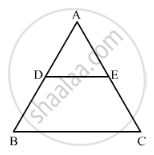Exercise 7.6 | Q 8.2 | Page 95

In Figure, DE || BC If DE = 4cm, BC = 8 cm and Area (ΔADE) = 25 cm2, find the area of ΔABC.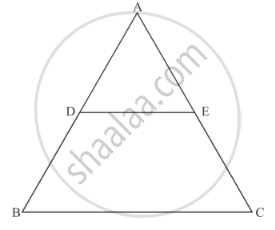Exercise 7.6 | Q 8.3 | Page 95

In the given figure, DE || BC and DE : BC = 3 : 5. Calculate the ratio of the areas of ∆ADE and the trapezium BCED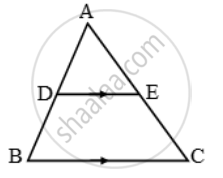Exercise 7.6 | Q 9 | Page 95

In ΔABC, D and E are the mid-points of AB and AC respectively. Find the ratio of the areas of ΔADE and ΔABC

Exercise 7.6 | Q 10 | Page 95

The areas of two similar triangles are 100 cm2 and 49 cm2 respectively. If the altitude the bigger triangle is 5 cm, find the corresponding altitude of the other.

Exercise 7.6 | Q 11 | Page 96

The areas of two similar triangles are 121 cm2 and 64 cm2 respectively. If the median of the first triangle is 12.1 cm, find the corresponding median of the other.

Exercise 7.6 | Q 12 | Page 96

If ΔABC ~ ΔDEF such that AB = 5 cm, area (ΔABC) = 20 cm2 and area (ΔDEF) = 45 cm2, determine DE.

Exercise 7.6 | Q 13 | Page 96

In ΔABC, PQ is a line segment intersecting AB at P and AC at Q such that PQ || BC and PQ divides ΔABC into two parts equal in area. Find (BP)/(AB)

Exercise 7.6 | Q 14 | Page 96

The areas of two similar triangles ABC and PQR are in the ratio 9:16. If BC = 4.5 cm, find the length of QR.

Exercise 7.6 | Q 15 | Page 96

ABC is a triangle and PQ is a straight line meeting AB in P and AC in Q. If AP = 1 cm, PB = 3 cm, AQ = 1.5 cm, QC = 4.5 m, prove that area of ΔAPQ is one- sixteenth of the area of ABC.

Exercise 7.6 | Q 18 | Page 96

Two isosceles triangles have equal vertical angles and their areas are in the ratio 36 : 25. Find the ratio of their corresponding heights.

Exercise 7.6 | Q 19 | Page 96

In Figure, ABC and DBC are two triangles on the same base BC. If AD intersects BC at O, show that (ar(ABC))/(ar(DBC)) = (AO)/(DO)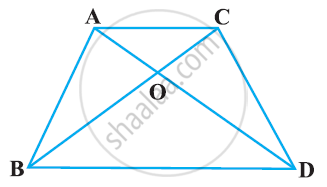Exercise 7.6 | Q 20 | Page 96

ABCD is a trapezium in which AB || CD. The diagonals AC and BD intersect at O. Prove that: (i) ΔAOB and ΔCOD (ii) If OA = 6 cm, OC = 8 cm,

Find:(a)  ("area"(triangleAOB))/("area"(triangleCOD))

(b)  ("area"(triangleAOD))/("area"(triangleCOD))

Exercise 7.6 | Q 20 | Page 96

If D is a point on the side AB of ΔABC such that AD : DB = 3.2 and E is a Point on BC such that DE || AC. Find the ratio of areas of ΔABC and ΔBDE.

Exercise 7.6 | Q 21 | Page 96

In ABC, P divides the side AB such that AP : PB = 1 : 2. Q is a point in AC such that PQ || BC. Find the ratio of the areas of ΔAPQ and trapezium BPQC.

Exercise 7.6 | Q 21 | Page 96

If ΔABC and ΔBDE are equilateral triangles, where D is the mid-point of BC, find the ratio of areas of ΔABC and ΔBDE.

Exercise 7.6 | Q 22 | Page 96

AD is an altitude of an equilateral triangle ABC. On AD as base, another equilateral triangle ADE is constructed. Prove that Area (ΔADE): Area (ΔABC) = 3: 4

Exercise 7.7 [Pages 119 - 121]

### RD Sharma solutions for Class 10 Maths Chapter 7 Triangles Exercise 7.7 [Pages 119 - 121]

Exercise 7.7 | Q 1 | Page 119

If the sides of a triangle are 3 cm, 4 cm, and 6 cm long, determine whether the triangle is a right-angled triangle.

Exercise 7.7 | Q 2.1 | Page 119

The sides of triangle is given below. Determine it is right triangle or not.

a = 7 cm, b = 24 cm and c = 25 cm

Exercise 7.7 | Q 2.2 | Page 119

The sides of triangle is given below. Determine it is right triangle or not.

a = 9 cm, b = l6 cm and c = 18 cm

Exercise 7.7 | Q 2.3 | Page 119

The sides of triangle is given below. Determine it is right triangle or not.

a = 1.6 cm, b = 3.8 cm and c = 4 cm

Exercise 7.7 | Q 2.4 | Page 119

The sides of triangle is given below. Determine it is right triangle or not.

a = 8 cm, b = 10 cm and c = 6 cm

Exercise 7.7 | Q 3 | Page 119

A man goes 15 metres due west and then 8 metres due north. How far is he from the starting point?

Exercise 7.7 | Q 4 | Page 119

A ladder 17 m long reaches a window of a building 15 m above the ground. Find the distance of the foot of the ladder from the building.

Exercise 7.7 | Q 5 | Page 119

Two poles of heights 6 m and 11 m stand on a plane ground. If the distance between the feet of the poles is 12 m, find the distance between their tops.

Exercise 7.7 | Q 6 | Page 120

In an isosceles triangle ABC, AB = AC = 25 cm, BC = 14 cm. Calculate the altitude from A on BC.

Exercise 7.7 | Q 7 | Page 120

The foot of a ladder is 6 m away from a wall and its top reaches a window 8 m above the ground. If the ladder is shifted in such a way that its foot is 8 m away from the wall, to what height does its tip reach?

Exercise 7.7 | Q 8 | Page 120

Two poles of height 9 m and 14 m stand on a plane ground. If the distance between their feet is 12 m, find the distance between their tops.

Exercise 7.7 | Q 9 | Page 120

Using Pythagoras theorem determine the length of AD in terms of b and c shown in Figure.

Exercise 7.7 | Q 10 | Page 120

A triangle has sides 5 cm, 12 cm and 13 cm. Find the length to one decimal place, of the perpendicular from the opposite vertex to the side whose length is 13 cm.

Exercise 7.7 | Q 11 | Page 120

ABCD is a square. F is the mid-point of AB. BE is one third of BC. If the area of ΔFBE = 108 cm2, find the length of AC.

Exercise 7.7 | Q 12 | Page 120

In an isosceles triangle ABC, if AB = AC = 13 cm and the altitude from A on BC is 5 cm, find BC.

Exercise 7.7 | Q 13 | Page 120

In a ΔABC, AB = BC = CA = 2a and AD ⊥ BC. Prove that

(i) AD = asqrt3

(ii) Area (ΔABC) = sqrt3 a2

Exercise 7.7 | Q 14 | Page 120

The lengths of the diagonals of a rhombus are 24 cm and 10 cm. Find each side of the rhombus.

Exercise 7.7 | Q 15 | Page 120

Each side of a rhombus is 10 cm. If one of its diagonals is 16 cm find the length of the other diagonal.

Exercise 7.7 | Q 16 | Page 120

Calculate the height of an equilateral triangle each of whose sides measures 12 cm.

Exercise 7.7 | Q 17 | Page 120

In the given figure, ∠B < 90° and segment AD ⊥ BC, show that

(i) b= h+ a+ x- 2ax

(ii) b2 = a2 + c2 - 2ax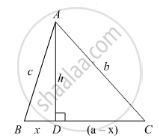Exercise 7.7 | Q 18

In an equilateral ΔABC, AD ⊥ BC, prove that AD2 = 3BD2.

Exercise 7.7 | Q 19 | Page 121

∆ABD is a right triangle right-angled at A and AC ⊥ BD. Show that

(i) AB2 = BC x BD

(ii) AC2 = BC x DC

(iii) AD2 = BD x CD

(iv) "AB"^2/"AC"^2="BD"/"DC"

Exercise 7.7 | Q 20 | Page 121

A guy wire attached to a vertical pole of height 18 m is 24 m long and has a stake attached to the other end. How far from the base of the pole should the stake be driven so that the wire will be taut?

Exercise 7.7 | Q 21 | Page 121

Determine whether the triangle having sides (a − 1) cm, 2sqrta cm and (a + 1) cm is a right-angled
triangle.

Exercise 7.7 | Q 22 | Page 121

In an acute-angled triangle, express a median in terms of its sides.

Exercise 7.7 | Q 23

In a quadrilateral ABCD, ∠B = 90°, AD2 = AB2 + BC2 + CD2, prove that ∠ACD = 90°.

Exercise 7.7 | Q 23 | Page 121

In right-angled triangle ABC in which ∠C = 90°, if D is the mid-point of BC, prove that AB2 = 4AD2 − 3AC2.

Exercise 7.7 | Q 24 | Page 121

In Figure, D is the mid-point of side BC and AE ⊥ BC. If BC = a, AC = b, AB = c, ED
= x, AD = p and AE = h, prove that:

(i) b^2 = p^2 + ax + a^2/4

(ii) c^2 = p^2 - ax + a^2/4

(iii) b^2 + c^2 = 2p^2 + a^2/2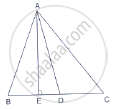Exercise 7.7 | Q 25 | Page 121

In ∆ABC, ∠A is obtuse, PB ⊥ AC and QC ⊥ AB. Prove that:

(i) AB ✕ AQ = AC ✕ AP

(ii) BC2 = (AC ✕ CP + AB ✕ BQ)

Exercise 7.7 | Q 26 | Page 121

In a right ∆ABC right-angled at C, if D is the mid-point of BC, prove that BC2 = 4(AD2 − AC2).

Exercise 7.7 | Q 28 | Page 121

An aeroplane leaves an airport and flies due north at a speed of 1000km/hr. At the same time, another aeroplane leaves the same airport and flies due west at a speed of 1200 km/hr. How far apart will be the two planes after 1 hours?

Exercise 7.8 [Pages 123 - 128]

### RD Sharma solutions for Class 10 Maths Chapter 7 Triangles Exercise 7.8 [Pages 123 - 128]

Exercise 7.8 | Q 1.1 | Page 123

In each of the figures [(i)-(iv)] given below, a line segment is drawn parallel to one side of the triangle and the lengths of certain line-segment are marked. Find the value of x in each of the following :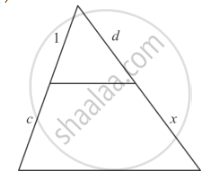Exercise 7.8 | Q 1.2 | Page 123

In each of the figures [(i)-(iv)] given below, a line segment is drawn parallel to one side of the triangle and the lengths of certain line-segment are marked. Find the value of x in each of the following :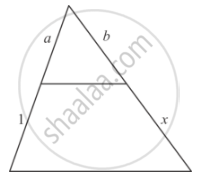Exercise 7.8 | Q 1.3 | Page 123

In each of the figures [(i)-(iv)] given below, a line segment is drawn parallel to one side of the triangle and the lengths of certain line-segment are marked. Find the value of x in each of the following :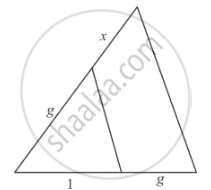Exercise 7.8 | Q 1.4 | Page 123

In each of the figures [(i)-(iv)] given below, a line segment is drawn parallel to one side of the triangle and the lengths of certain line-segment are marked. Find the value of x in each of the following :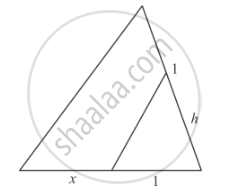Exercise 7.8 | Q 2 | Page 123

What values of x will make DE || AB in the given figure?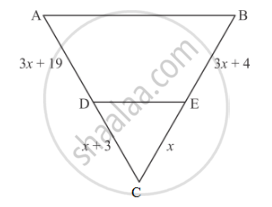Exercise 7.8 | Q 3 | Page 123

In ∆ABC, points P and Q are on CA and CB, respectively such that CA = 16 cm, CP = 10 cm, CB = 30 cm and CQ = 25 cm. Is PQ || AB?

Exercise 7.8 | Q 4 | Page 123

In the given figure, DE || BD. Determine AC and AE.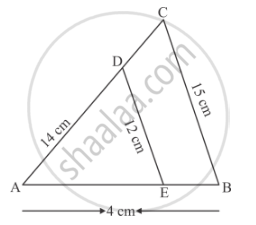Exercise 7.8 | Q 5.1 | Page 124

In the given figure, given that ∆ABC ∼ ∆PQR and quad ABCD ∼ quad PQRS. Determine the value of xy, z in each case.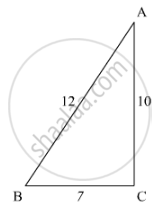Exercise 7.8 | Q 5.2 | Page 124

In the given figure, given that ∆ABC ∼ ∆PQR and quad ABCD ∼ quad PQRS. Determine the value of xy, z in each case.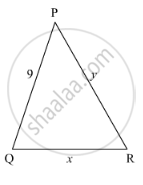Exercise 7.8 | Q 6 | Page 124

In ∆ABC, P and Q are points on sides AB and AC respectively such that PQ || BC. If AP = 4 cm, PB = 6 cm and PQ = 3 cm, determine BC.

Exercise 7.8 | Q 7.1 | Page 124

In each of the following figures, you find who triangles. Indicate whether the triangles are similar. Give reasons in support of your answer.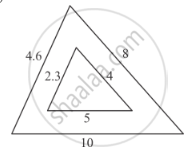Exercise 7.8 | Q 7.2 | Page 124

In each of the following figures, you find who triangles. Indicate whether the triangles are similar. Give reasons in support of your answer.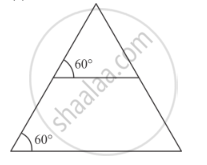Exercise 7.8 | Q 7.3 | Page 124

In each of the following figures, you find who triangles. Indicate whether the triangles are similar. Give reasons in support of your answer.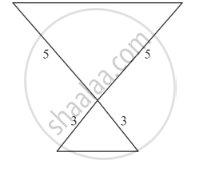Exercise 7.8 | Q 7.4 | Page 124

In each of the following figures, you find who triangles. Indicate whether the triangles are similar. Give reasons in support of your answer.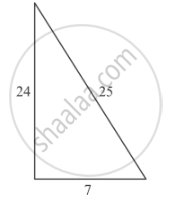Exercise 7.8 | Q 7.5 | Page 124

In each of the following figures, you find who triangles. Indicate whether the triangles are similar. Give reasons in support of your answer.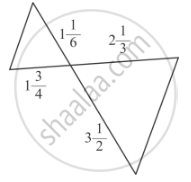Exercise 7.8 | Q 8 | Page 125

In ∆PQR, M and N are points on sides PQ and PR respectively such that PM = 15 cm and NR = 8 cm. If PQ = 25 cm and PR = 20 cm state whether MN || QR.

Exercise 7.8 | Q 9 | Page 125

In ∆ABC, P and Q are points on sides AB and AC respectively such that PQ || BC. If AP = 3 cm, PB = 5 cm and AC = 8 cm, find AQ.

Exercise 7.8 | Q 10 | Page 125

In the given figure, ∆AMB ∼ ∆CMD; determine MD in terms of xy and z.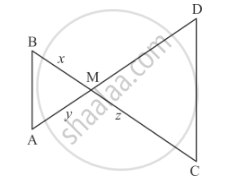Exercise 7.8 | Q 11 | Page 125

In ∆ABC, the bisector of ∠A intersects BC in D. If AB = 18 cm, AC = 15 cm and BC = 22 cm, find BD.

Exercise 7.8 | Q 12 | Page 125

In the given figure, l || m

(i) Name three pairs of similar triangles with proper correspondence; write similarities.
(ii) Prove that

$\frac{AB}{PQ} = \frac{AC}{PR} = \frac{BC}{RQ}$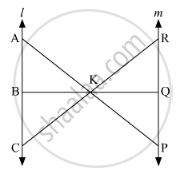Exercise 7.8 | Q 13.1 | Page 125

In the given figure,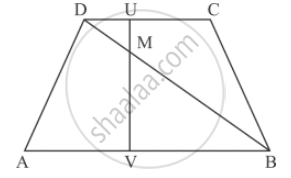AB || DC prove that

(i) ∆DMU ∼ ∆BMV

Exercise 7.8 | Q 13.2 | Page 125

In the given figure,AB || DC prove that

DM × BV = BM ✕ DU

Exercise 7.8 | Q 14 | Page 126

ABCD is a trapezium in which AB || DC. P and Q are points on sides AD and BC such that PQ || AB. If PD = 18, BQ = 35 and QC = 15, find AD.

Exercise 7.8 | Q 15 | Page 126

In ∆ABC, D and E are points on sides AB and AC respectively such that AD ✕ EC = AE ✕ DB. Prove that DE || BC.

Exercise 7.8 | Q 16 | Page 126

ABCD is a trapezium having AB || DC. Prove that O, the point of intersection of diagonals, divides the two diagonals in the same ratio. Also prove that

$\frac{ar\left( ∆ OCD \right)}{ar\left( ∆ OAB \right)} = \frac{1}{9}$ if AB = 3 CD.
Exercise 7.8 | Q 17 | Page 126

Corresponding sides of two triangles are in the ratio 2 : 3. If the area of the smaller triangle is 48 cm2, determine the area of the larger triangle.

Exercise 7.8 | Q 18 | Page 126

The area of two similar triangles are 36 cm2 and 100 cm2. If the length of a side of the smaller triangle in 3 cm, find the length of the corresponding side of the larger triangle.

Exercise 7.8 | Q 19 | Page 126

Corresponding sides of two similar triangles are in the ratio 1 : 3. If the area of the smaller triangle in 40 cm2, find the area of the larger triangle.

Exercise 7.8 | Q 20 | Page 126

In the given figure, each of PA, QB, RC and SD is perpendicular to l. If AB = 6 cm, BC = 9 cm, CD = 12 cm and PS = 36 cm, then determine PQ, QR and RS.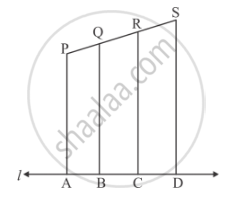Exercise 7.8 | Q 21.1 | Page 126

In each of the figures given below, an altitude is drawn to the hypotenuse by a right-angled triangle. The length of different line-segment are marked in each figure. Determine xyz in each case.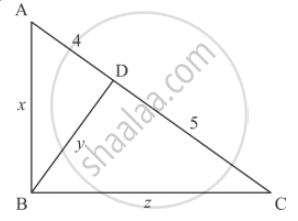Exercise 7.8 | Q 21.2 | Page 126

In each of the figures given below, an altitude is drawn to the hypotenuse by a right-angled triangle. The length of different line-segment are marked in each figure. Determine x, y, z in each case.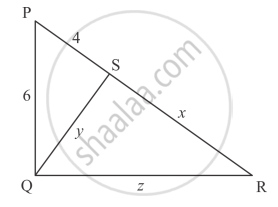Exercise 7.8 | Q 22 | Page 127

Prove that in an equilateral triangle, three times the square of a side is equal to four times the square of its altitudes.

Exercise 7.8 | Q 23 | Page 127

In ∆ABC, AD and BE are altitude. Prove that$\frac{ar\left( ∆ DEC \right)}{ar\left( ∆ ABC \right)} = \frac{{DC}^2}{{AC}^2}$

Exercise 7.8 | Q 24 | Page 127

The diagonals of quadrilateral ABCD intersect at O. Prove that
[A(∆"ACB")]/[A(∆"ACD")] = "BO"/"DO"

Exercise 7.8 | Q 25.1 | Page 127

In ∆ABC, ray AD bisects ∠A and intersects BC in D. If BC = a, AC = b and AC = c, prove that $BD = \frac{ac}{b + c}$

Exercise 7.8 | Q 25.2 | Page 127

In ∆ABC, ray AD bisects ∠A and intersects BC in D. If BC = a, AC = b and AC = c, prove that $DC = \frac{ab}{b + c}$

Exercise 7.8 | Q 26 | Page 127

There is a staircase as shown in the given figure, connecting points A and B. Measurements of steps are marked in the figure. Find the straight line distance between A and B.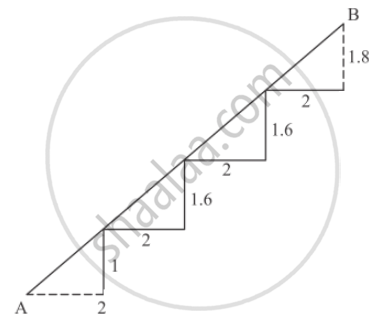Exercise 7.8 | Q 27 | Page 127

In ∆ABC, ∠A = 60°. Prove that BC2 = AB2 + AC2 − AB . AC.

Exercise 7.8 | Q 28 | Page 127

In ∆ABC, ∠C is an obtuse angle. AD ⊥ BC and AB2 = AC2 + 3 BC2. Prove that BC = CD.

Exercise 7.8 | Q 29 | Page 127

A point D is on the side BC of an equilateral triangle ABC such that$DC = \frac{1}{4}BC$. Prove that AD2 = 13 CD2.

Exercise 7.8 | Q 30 | Page 127

In ∆ABC, if BD ⊥ AC and BC2 = 2 AC . CD, then prove that AB = AC.

Exercise 7.8 | Q 31 | Page 127

In a quadrilateral ABCD, given that ∠A + ∠D = 90°. Prove that AC2 + BD2 = AD2 + BC2.

Exercise 7.8 | Q 32 | Page 127

In ∆ABC, given that AB AC and BD ⊥ AC. Prove that BC2 = 2 ACCD

Exercise 7.8 | Q 33 | Page 127

ABCD is a rectangle. Points M and N are on BD such that AM ⊥ BD and CN  ⊥ BD. Prove that BM2 + BN2 = DM2 + DN2.

Exercise 7.8 | Q 34 | Page 127

In ∆ABC, AD is a median. Prove that AB2 + AC2 = 2AD2 + 2DC2.

Exercise 7.8 | Q 35 | Page 127

In ∆ABC, ∠ABC = 135°. Prove that AC2 = AB2 + BC2 + 4 ar (∆ABC)

Exercise 7.8 | Q 36 | Page 127

In a quadrilateral ABCD, ∠B = 90°. If AD2 = AB2 + BC2 + CD2 then prove that ∠ACD = 90°.

Exercise 7.8 | Q 37 | Page 127

In a triangle ABC, N is a point on AC such that BN ⊥ AC. If BN2 = AN . NC, prove that ∠B = 90°.

Exercise 7.8 | Q 38 | Page 128

Nazima is fly fishing in a stream. The tip of her fishing rod is 1.8 m above the surface of the water and the fly at the end of the string rests on the water 3.6 m away and 2.4 m from a point directly under the tip of the road. Assuming that her string (from the tip of her road to the fly) is taut, how much string does she have out (in the given figure)? If she pulls the string at the rate of 5 cm per second, what will the horizontal distance of the fly from her after 12 seconds.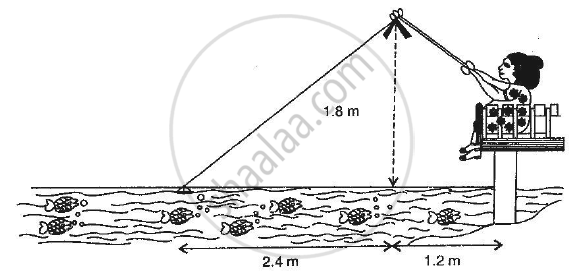Exercise 7.9 [Pages 128 - 130]

### RD Sharma solutions for Class 10 Maths Chapter 7 Triangles Exercise 7.9 [Pages 128 - 130]

Exercise 7.9 | Q 1 | Page 128

State basic proportionality theorem and its converse.

Exercise 7.9 | Q 2 | Page 128

In the adjoining figure, find AC.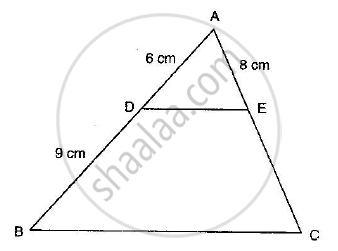Exercise 7.9 | Q 3 | Page 129

In the adjoining figure, if AD is the bisector of ∠A, what is AC?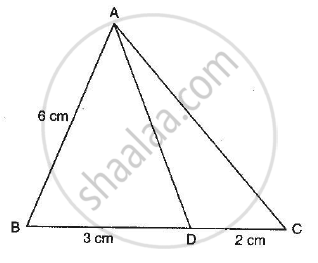Exercise 7.9 | Q 4 | Page 129

State AAA similarity criterion.

Exercise 7.9 | Q 5 | Page 129

State SSS similarity criterion.

Exercise 7.9 | Q 6 | Page 129

State SAS similarity criterion.

Exercise 7.9 | Q 7 | Page 129

In the adjoining figure, DE is parallel to BC and AD = 1 cm, BD = 2 cm. What is the ratio of the area of ∆ABC to the area of ∆ADE?

Exercise 7.9 | Q 8 | Page 129

In the figure given below DE || BC. If AD = 2.4 cm, DB = 3.6 cm, AC = 5 cm. Find AE.

Exercise 7.9 | Q 9 | Page 129

If the areas of two similar triangles ABC and PQR are in the ratio 9 : 16 and BC = 4.5 cm, what is the length of QR?

Exercise 7.9 | Q 10 | Page 129

The areas of two similar triangles are 169 cm2 and 121 cm2 respectively. If the longest side of the larger triangle is 26 cm, what is the length of the longest side of the smaller triangle?

Exercise 7.9 | Q 11 | Page 129

If ABC and DEF are similar triangles such that ∠A = 57° and ∠E = 73°, what is the measure of ∠C?

Exercise 7.9 | Q 12 | Page 129

If the altitude of two similar triangles are in the ratio 2 : 3, what is the ratio of their areas?

Exercise 7.9 | Q 13 | Page 129

If ∆ABC and ∆DEF are two triangles such that$\frac{AB}{DE} = \frac{BC}{EF} = \frac{CA}{FD} = \frac{3}{4}$, then write Area (∆ABC) : Area (∆DEF)

Exercise 7.9 | Q 14 | Page 129

If ∆ABC and ∆DEF are similar triangles such that AB = 3 cm, BC = 2 cm, CA = 2.5 cm and EF = 4 cm, write the perimeter of ∆DEF.

Exercise 7.9 | Q 15 | Page 129

State Pythagoras theorem and its converse.

Exercise 7.9 | Q 16 | Page 129

The lengths of the diagonals of a rhombus are 30 cm and 40 cm. Find the side of the rhombus.

Exercise 7.9 | Q 17 | Page 130

In the given figure, PQ || BC and AP : PB = 1 : 2. Find$\frac{area \left( ∆ APQ \right)}{area \left( ∆ ABC \right)}$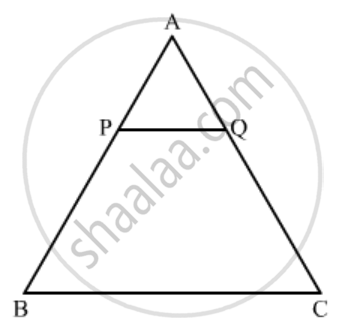Exercise 7.9 | Q 18 | Page 130

In the given figure, S and T are points on the sides PQ and PR respectively of ∆PQR such that PT = 2 cm, TR = 4 cm and ST is parallel to QR. Find the ratio of the areas of ∆PST and ∆PQR.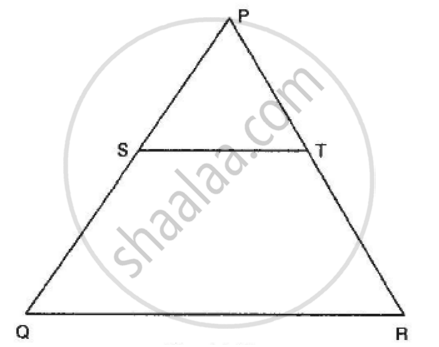Exercise 7.9 | Q 19 | Page 130

In the given figure, ∆AHK is similar to ∆ABC. If AK = 10 cm, BC = 3.5 cm and HK = 7 cm, find AC.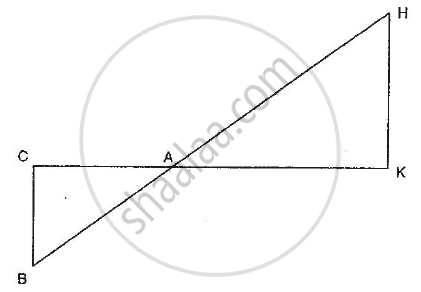Exercise 7.9 | Q 20 | Page 130

In the given figure, DE || BC in ∆ABC such that BC = 8 cm, AB = 6 cm and DA = 1.5 cm. Find DE.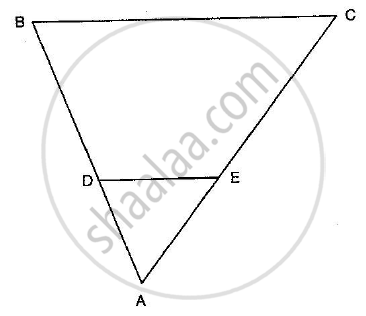Exercise 7.9 | Q 21 | Page 131

In the given figure, DE || BC and $AD = \frac{1}{2}BD$. If BC = 4.5 cm, find DE.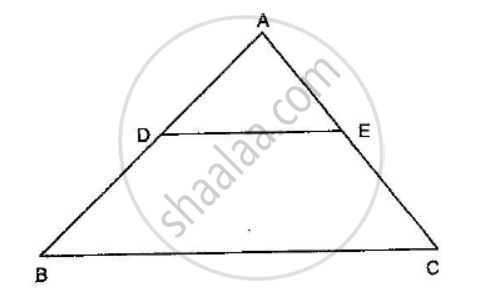Exercise 7.9 | Q 22 | Page 130

In the given figure, LM = LN = 46°. Express x in terms of aand c where abc are lengths of LM, MN and NK respectively.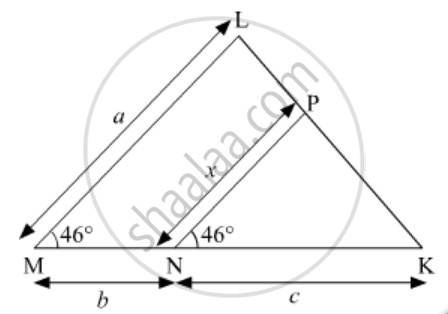Exercise 7.10 [Pages 131 - 136]

### RD Sharma solutions for Class 10 Maths Chapter 7 Triangles Exercise 7.10 [Pages 131 - 136]

Exercise 7.10 | Q 1 | Page 131

Sides of two similar triangles are in the ratio 4 : 9. Areas of these triangles are in the ratio.

• 2 : 3

• 4 : 9

• 81 : 16

• 16 : 81

Exercise 7.10 | Q 2 | Page 131

The areas of two similar triangles are in respectively 9 cm2 and 16 cm2. The ratio of their corresponding sides is

•  3 : 4

• 4 : 3

•  2 : 3

• 4 : 5

Exercise 7.10 | Q 3 | Page 131

The areas of two similar triangles ∆ABC and ∆DEF are 144 cm2 and 81 cm2 respectively. If the longest side of larger ∆ABC be 36 cm, then the longest side of the smaller triangle ∆DEF is

• 20 cm

• 26 cm

• 27 cm

• 30 cm

Exercise 7.10 | Q 4 | Page 132

∆ABC and ∆BDE are two equilateral triangles such that D is the mid-point of BC. The ratio of the areas of triangle ABC and BDE is

• 2 : 1

• 1 : 2

• 4 : 1

• 1 : 4

Exercise 7.10 | Q 5 | Page 132

If ∆ABC and ∆DEF are similar such that 2AB = DE and BC = 8 cm, then EF =

• 16 cm

• 12 cm

• 8 cm

• 4 cm

Exercise 7.10 | Q 6 | Page 132

If ∆ABC and ∆DEF are two triangles such tha$\frac{AB}{DE} = \frac{BC}{EF} = \frac{CA}{FD} = \frac{2}{5}$ , then Area (∆ABC) : Area (∆DEF) =

• 2:5

• 4 : 25

• 4 : 15

• 8 : 125

Exercise 7.10 | Q 7 | Page 132

XY is drawn parallel to the base BC of a ∆ABC cutting AB at X and AC at Y. If AB = 4 BX and YC = 2 cm, then AY =

• 2 cm

• 4 cm

• 6 cm

• 8 cm

Exercise 7.10 | Q 8 | Page 132

Two poles of height 6 m and 11 m stand vertically upright on a plane ground. If the distance between their foot is 12 m, the distance between their tops is

• 12 m

•  14 m

• 13 m

• 11 m

Exercise 7.10 | Q 9 | Page 132

In ∆ABC, D and E are points on side AB and AC respectively such that DE || BC and AD : DB = 3 : 1. If EA = 3.3 cm, then AC =

• 1.1 cm

• 4 cm

• 4.4 cm

• 5.5 cm

Exercise 7.10 | Q 10 | Page 132

In triangles ABC and DEF, ∠A = ∠E = 40°, AB : ED = AC : EF and ∠F = 65°, then ∠B =

• 35°

• 65°

•  75°

• 85°

Exercise 7.10 | Q 11 | Page 132

If ABC and DEF are similar triangles such that ∠A = 47° and ∠E = 83°, then ∠C =

• 50°

•  60°

• 70°

• 80°

Exercise 7.10 | Q 12 | Page 132

If D, E, F are the mid-points of sides BC, CA and AB respectively of ∆ABC, then the ratio of the areas of triangles DEF and ABC is

• 1 : 4

• 1 : 2

• 2 : 3

• 4 : 5

Exercise 7.10 | Q 13 | Page 132

In a ∆ABC, ∠A = 90°, AB = 5 cm and AC = 12 cm. If AD ⊥ BC, then AD =

• $\frac{13}{2}cm$

• $\frac{60}{13}cm$
• $\frac{13}{60}cm$
• $\frac{2\sqrt{15}}{13}cm$
Exercise 7.10 | Q 14 | Page 132

If ∆ABC is an equilateral triangle such that AD ⊥ BC, then AD2 =

• $\frac{3}{2} {DC}^2$
• 2 DC2

• 3 CD2

• 4 DC2

Exercise 7.10 | Q 15 | Page 132

In a ∆ABC, AD is the bisector of ∠BAC. If AB = 6 cm, AC = 5 cm and BD = 3 cm, then DC =

• 11.3 cm

• 2.5 cm

•  3 : 5 cm

• None of these

Exercise 7.10 | Q 16 | Page 132

In a ∆ABC, AD is the bisector of ∠BAC. If AB = 8 cm, BD = 6 cm and DC = 3 cm. Find AC

•  4 cm

•  6 cm

• 3 cm

• 8 cm

Exercise 7.10 | Q 17 | Page 132

ABCD is a trapezium such that BC || AD and AD = 4 cm. If the diagonals AC and BD intersect at O such that $\frac{AO}{OC} = \frac{DO}{OB} = \frac{1}{2}$, then BC =

• 7 cm

• 8 cm

• 9 cm

• 6 cm

Exercise 7.10 | Q 18 | Page 133

If ABC is a right triangle right-angled at B and M, N are the mid-points of AB and BC respectively, then 4(AN2 + CM2) =

• 4 AC2

•  5 AC2

• $\frac{5}{4} {AC}^2$
• 6 AC2

Exercise 7.10 | Q 19 | Page 133

If in ∆ABC and ∆DEF, $\frac{AB}{DE} = \frac{BC}{FD}$, then ∆ABC ∼ ∆DEF when

• ∠A = ∠F

• ∠A = ∠D

• ∠B = ∠D

• ∠B = ∠E

Exercise 7.10 | Q 20 | Page 133

If in two triangles ABC and DEF, $\frac{AB}{DE} = \frac{BC}{FE} = \frac{CA}{FD}$, then

• ∆FDE ∼ ∆CAB

• ∆FDE ∼ ∆ABC

• ∆CBA ∼ ∆FDE

• ∆BCA ∼ ∆FDE

Exercise 7.10 | Q 21 | Page 133

∆ABC ∼ ∆DEF, ar(∆ABC) = 9 cm2, ar(∆DEF) = 16 cm2. If BC = 2.1 cm, then the measure of EF is

• 2.8 cm

• 4.2 cm

•  2.5 cm

• 4.1 cm

Exercise 7.10 | Q 22 | Page 133

The length of the hypotenuse of an isosceles right triangle whose one side is$4\sqrt{2} cm$

• 12 cm

• 8 cm

• $8\sqrt{2} cm$
• $12\sqrt{2} cm$
Exercise 7.10 | Q 23 | Page 136

In a ∆ABC, perpendicular AD from A and BC meets BC at D. If BD = 8 cm, DC = 2 cm and AD = 4 cm, then

•  ∆ABC is isosceles

• ∆ABC is equilateral

• AC = 2AB

• ∆ABC is right-angled at A

Exercise 7.10 | Q 23 | Page 133

A man goes 24 m due west and then 7 m due north. How far is he from the starting point?

• 31 m

• 17 m

• 25 m

• 26 m

Exercise 7.10 | Q 24 | Page 133

∆ABC ∼ ∆DEF. If BC = 3 cm, EF = 4 cm and ar(∆ABC) = 54 cm2, then ar(∆DEF) =

•  108 cm2

• 96 cm2

• 48 cm2

• 100 cm2

Exercise 7.10 | Q 25 | Page 133

∆ABC ∼ ∆PQR such that ar(∆ABC) = 4 ar(∆PQR). If BC = 12 cm, then QR =

• 9 cm

• 10 cm

• 6 cm

• 8 cm

Exercise 7.10 | Q 26 | Page 133

The areas of two similar triangles are 121 cm2 and 64 cm2 respectively. If the median of the first triangle is 12.1 cm, then the corresponding median of the other triangle is

• 11 cm

•  8.8 cm

• 11.1 cm

• 8.1 cm

Exercise 7.10 | Q 27 | Page 133

In an equilateral triangle ABC if AD ⊥ BC, then AD2 =

•  CD2

• 2CD2

• 3CD2

• 4CD2

Exercise 7.10 | Q 29 | Page 133

In an equilateral triangle ABC if AD ⊥ BC, then

Exercise 7.10 | Q 30 | Page 133

∆ABC is an isosceles triangle in which ∠C = 90. If AC = 6 cm, then AB =

• $6\sqrt{2} cm$
• 6 cm

• $2\sqrt{6} cm$
• $4\sqrt{2} cm$
Exercise 7.10 | Q 31 | Page 133

If in two triangle ABC and DEF, ∠A = ∠E, ∠B = ∠F, then which of the following is not true?

(a)$\frac{BC}{DF} = \frac{AC}{DE}$

(b)$\frac{AB}{DE} = \frac{BC}{DF}$

(c)$\frac{AB}{EF} = \frac{AC}{DE}$

(d)$\frac{BC}{DF} = \frac{AB}{EF}$

• $\frac{BC}{DF} = \frac{AC}{DE}$
• $\frac{AB}{DE} = \frac{BC}{DF}$
• $\frac{AB}{EF} = \frac{AC}{DE}$
• $\frac{BC}{DF} = \frac{AB}{EF}$
Exercise 7.10 | Q 32 | Page 133

In the given figure the measure of ∠D and ∠F are respectively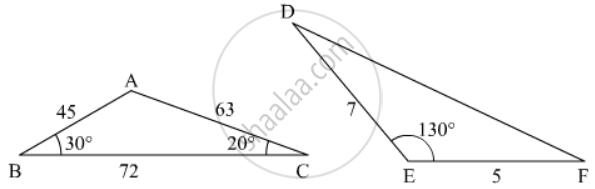• 50°, 40°

• 20°, 30°

•  40°, 50°

• 30°, 20°

Exercise 7.10 | Q 33 | Page 134

In the given figure, the value of x for which DE || AB is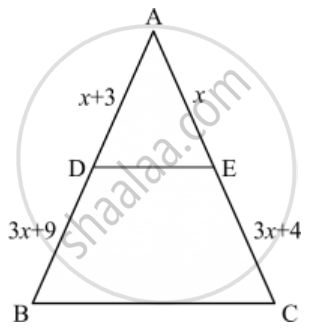• 4

• 1

• 3

• 2

Exercise 7.10 | Q 34 | Page 134

In the given figure, if ∠ADE = ∠ABC, then CE =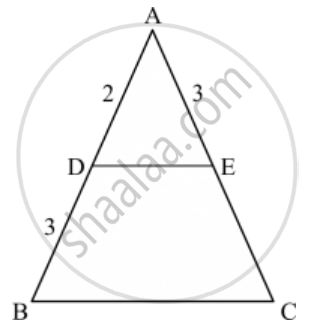• 2

• 5

• 9/2

• 3

Exercise 7.10 | Q 35 | Page 134

In the given figure, RS || DB || PQ. If CP = PD = 11 cm and DR = RA = 3 cm. Then the values of x and y are respectively.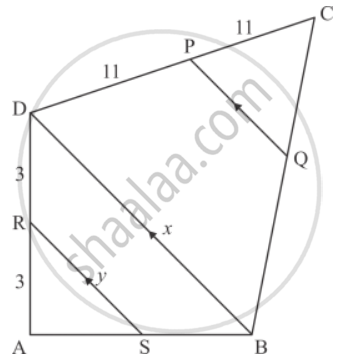• 12, 10

• 14, 6

•  10, 7

• 16, 8

Exercise 7.10 | Q 36 | Page 135

In the given figure, if PB || CF and DP || EF, then $\frac{AD}{DE} =$

• $\frac{3}{4}$
• $\frac{1}{3}$
• $\frac{1}{4}$
• $\frac{2}{3}$
Exercise 7.10 | Q 37 | Page 135

A chord of a circle of radius 10 cm subtends a right angle at the centre. The length of the chord (in cm) is

• $5\sqrt{2}$
• $10\sqrt{2}$
• $\frac{5}{\sqrt{2}}$
• $10\sqrt{3}$
Exercise 7.10 | Q 38 | Page 135

A vertical stick 20 m long casts a shadow 10 m long on the ground. At the same time, a tower casts a shadow 50 m long on the ground. The height of the tower is

•  100 m

• 120 m

• 25 m

• 200 m

Exercise 7.10 | Q 39 | Page 135

Two isosceles triangles have equal angles and their areas are in the ratio 16 : 25. The ratio of their corresponding heights is

• 4 : 5

• 5 : 4

• 3 : 2

• 5 : 7

Exercise 7.10 | Q 40 | Page 135

∆ABC is such that AB = 3 cm, BC = 2 cm and CA = 2.5 cm. If ∆DEF ∼ ∆ABC and EF = 4 cm, then perimeter of ∆DEF is

• 7.5 cm

• 15 cm

• 22.5 cm

• 30 cm

Exercise 7.10 | Q 41 | Page 136

In ∆ABC, a line XY parallel to BC cuts AB at X and AC at Y. If BY bisects ∠XYC, then

•  BC = CY

• BC = BY

•  BC ≠ CY

• BC ≠ BY

Exercise 7.10 | Q 42 | Page 136

In a ∆ABC, ∠A = 90°, AB = 5 cm and AC = 12 cm. If AD ⊥ BC, then AD =

• $\frac{13}{2}cm$
• $\frac{60}{13}cm$
• $\frac{13}{60}cm$
• $\frac{2\sqrt{15}}{13}cm$
Exercise 7.10 | Q 44 | Page 136

In a ∆ABC, point D is on side AB and point E is on side AC, such that BCED is a trapezium. If DE : BC = 3 : 5, then Area (∆ ADE) : Area (◻BCED) =

• 3 : 4

•  9 : 16

• 3 : 5

• 9 : 25

Exercise 7.10 | Q 45 | Page 136

If ABC is an isosceles triangle and D is a point of BC such that AD ⊥ BC, then

• AB2 − AD2 = BD.DC

• AB2 − AD2 = BD2 − DC2

• AB2 + AD2 = BD.DC

• AB2 + AD2 = BD2 − DC2

Exercise 7.10 | Q 46 | Page 136

∆ABC is a right triangle right-angled at A and  AD ⊥ BC. Then, $\frac{BD}{DC} =$

• $\left( \frac{AB}{AC} \right)^2$
• $\frac{AB}{AC}$
• $\left( \frac{AB}{AD} \right)^2$
• $\frac{AB}{AD}$
• Non of the above

Exercise 7.10 | Q 47 | Page 136

If E is a point on side CA of an equilateral triangle ABC such that BE ⊥ CA, then AB2 + BC2 + CA2 =

• 2 BE2

• 3 BE2

• 4 BE2

• 6 BE2

Exercise 7.10 | Q 48 | Page 136

In a right triangle ABC right-angled at B, if P and Q are points on the sides AB and AC respectively, then

• AQ2 + CP2 = 2(AC2 + PQ2)

• 2(AQ2 + CP2) = AC2 + PQ2

• AQ2 + CP2 = AC2 + PQ2

• $AQ + CP = \frac{1}{2}\left( AC + PQ \right)$

Exercise 7.10 | Q 49 | Page 136

If ∆ABC ∼ ∆DEF such that DE = 3 cm, EF = 2 cm, DF = 2.5 cm, BC = 4 cm, then perimeter of ∆ABC is

•  18 cm

• 20 cm

• 12 cm

• 15 cm

Exercise 7.10 | Q 50 | Page 136

If ∆ABC ∼ ∆DEF such that AB = 9.1 cm and DE = 6.5 cm. If the perimeter of ∆DEF is 25 cm, then the perimeter of ∆ABC is

• 36 cm

•  30 cm

• 34 cm

• 35 cm

Exercise 7.10 | Q 51 | Page 136

In an isosceles triangle ABC if AC = BC and AB2 = 2AC2, then ∠C =

• 30°

•  45°

• 90°

• 60°

## Chapter 7: Triangles

Exercise 7.1Exercise 7.2Exercise 7.3Exercise 7.4Exercise 7.5Exercise 7.6Exercise 7.7Exercise 7.8Exercise 7.9Exercise 7.10## RD Sharma solutions for Class 10 Maths chapter 7 - Triangles

RD Sharma solutions for Class 10 Maths chapter 7 (Triangles) include all questions with solution and detail explanation. This will clear students doubts about any question and improve application skills while preparing for board exams. The detailed, step-by-step solutions will help you understand the concepts better and clear your confusions, if any. Shaalaa.com has the CBSE Class 10 Maths solutions in a manner that help students grasp basic concepts better and faster.

Further, we at Shaalaa.com provide such solutions so that students can prepare for written exams. RD Sharma textbook solutions can be a core help for self-study and acts as a perfect self-help guidance for students.

Concepts covered in Class 10 Maths chapter 7 Triangles are Properties of Ratios of Areas of Two Triangles, Similar Triangles, Areas of Similar Triangles, Basic Proportionality Theorem (Thales Theorem), Property of an Angle Bisector of a Triangle, Property of Three Parallel Lines and Their Transversals, Converse of Basic Proportionality Theorem, Similarity of Triangles, Criteria for Similarity of Triangles, Similar Figures, Similarity of Triangles, Basic Proportionality Theorem (Thales Theorem), Criteria for Similarity of Triangles, Areas of Similar Triangles, Application of Pythagoras Theorem in Acute Angle and Obtuse Angle, Triangles Examples and Solutions, Angle Bisector, Ratio of Sides of Triangle, Right-angled Triangles and Pythagoras Property, Similarity of Triangles, Similarity of Triangles.

Using RD Sharma Class 10 solutions Triangles exercise by students are an easy way to prepare for the exams, as they involve solutions arranged chapter-wise also page wise. The questions involved in RD Sharma Solutions are important questions that can be asked in the final exam. Maximum students of CBSE Class 10 prefer RD Sharma Textbook Solutions to score more in exam.

Get the free view of chapter 7 Triangles Class 10 extra questions for Class 10 Maths and can use Shaalaa.com to keep it handy for your exam preparation